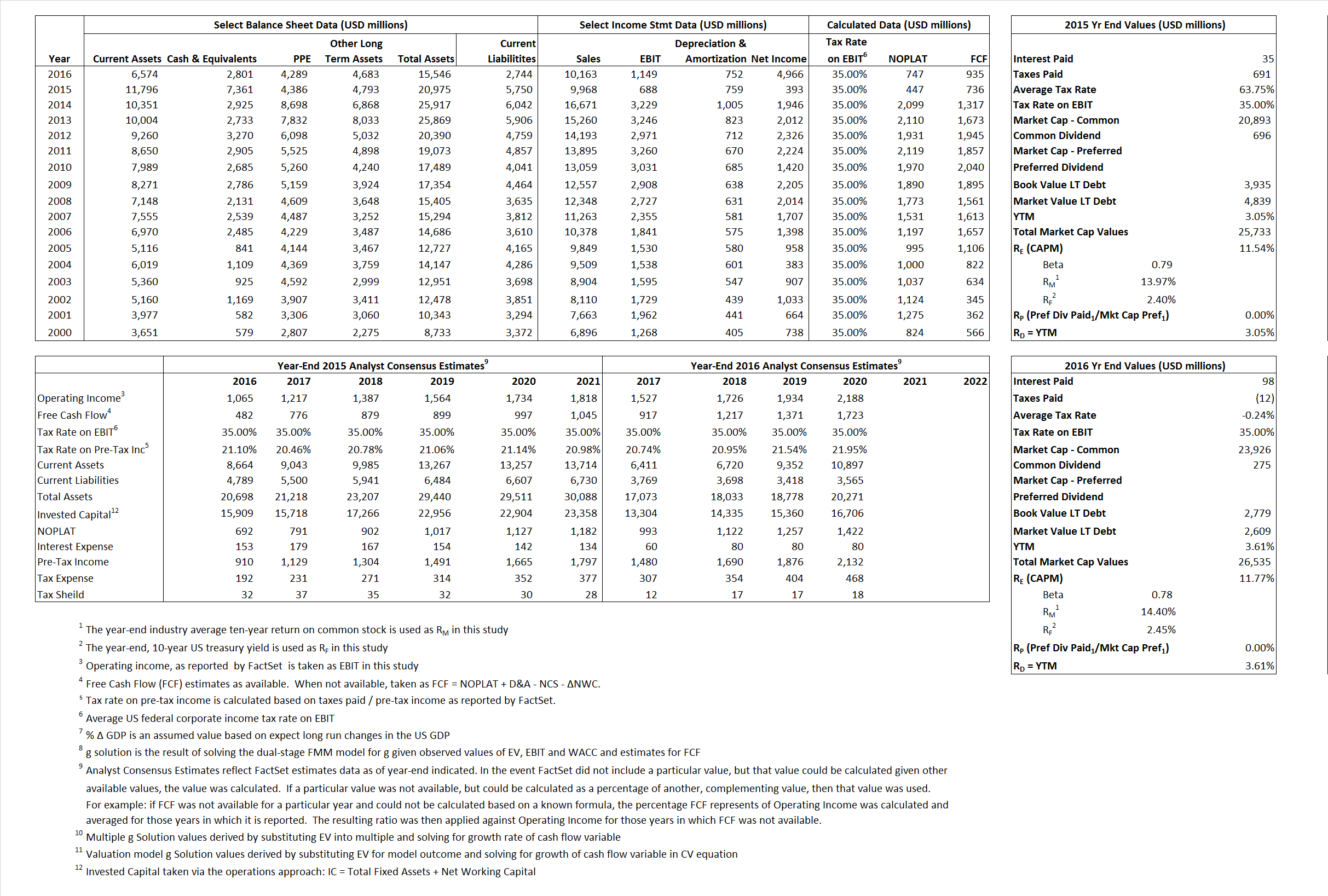# Baxter International Inc.

## Analyst Listing

The following analysts provide coverage for the subject firm as of May 2016:

 Broker Analyst Analyst Email HC Wainwright Andrew S. Fein afein@hwco.com Jefferies Brian Abrahams babrahams@jefferies.com Piper Jaffray Brooks E. West brooks.e.west@pjc.com Raymond James Christopher J. Raymond chris.raymond@raymondjames.com Leerink Partners Danielle Antalffy danielle.antalffy@leerink.com Cowen & Company Eric Schmidt eric.schmidt@cowen.com Leerink Partners Geoffrey C. Porges geoffrey.porges@leerink.com RBC Capital Markets Glenn Novarro glenn.novarro@rbccm.com BMO Capital Markets Joanne K. Wuensch joanne.wuensch@bmo.com William Blair John Sonnier jsonnier@williamblair.com Cowen & Company Joshua Jennings josh.jennings@cowen.com Piper Jaffray Joshua Schimmer joshua.e.schimmer@pjc.com Raymond James Lawrence S. Keusch lawrence.keusch@raymondjames.com BMO Capital Markets M. Ian Somaiya ian.somaiya@bmo.com William Blair Margaret Kaczor mkaczor@williamblair.com Evercore ISI Mark Schoenebaum mark.schoenebaum@evercoreisi.com Credit Suisse Matthew Keeler matthew.keeler@credit-suisse.com Keefe Bruyette & Woods Meyer Shields mshields@kbw.com RBC Capital Markets Michael J. Yee michael.yee@rbccm.com Stifel Nicolaus Rick Wise wiser@stifel.com Guggenheim Securities William Tanner btanner@fbr.com

## Primary Input Data## Derived Input Data

### Equational Form

Net Operating Profit Less Adjusted Taxes NOPLAT 447 747$NOPLAT\, =\, EBIT\, x\, (1 \,-\, Avg \,\,Tax\,\, Rate\,\, on\,\, EBIT)$
Free Cash Flow FCF 736 935$FCF\,=NOPLAT\,+\,Non-Cash\,Expenses-\Delta NWC\,-\,NCS$
Tax Shield TS 22 (0)$TS\,=\,Interest\,\,Paid\,\,x\,\, Avg \,\,Tax\,\,Rate\,\, on\,\, Pre-Tax\,\, Income$
Invested Capital IC 15,225 12,802$IC\,=\,Fixed\,\,Operating\,\,Assets\,\,+\,\,Net\,\, Working\,\, Capital$
Return on Invested Capital ROIC 2.94% 5.83%$ROIC\,=\,\frac { NOPLAT }{ IC }$
Net Investment NetInv (3,891) (1,671)$NetInv\,=\,{ {IC}_{1}}-{{IC}_{0}}+Depreciation$
Investment Rate IR -870.08% -223.74%$IR\,=\,\frac {NetInv}{NOPLAT}$
Weighted Average Cost of Capital
WACCMarket 9.58% 10.97%$WACC\,=\,\frac { E }{ V } { R }_{ E }\,+\,\frac { P }{ V } { R }_{ P }\,+\,\frac { D }{ V } { R }_{ D }\left( 1- Avg\,\, Tax\,\,Rate\,\,on\,\,Pre-Tax\,\,Income \right)$
WACCBook    6.54%    7.05%
Enterprise value
EVMarket  18,372  23,734$EV\,=\,Market\,\,Cap\,\,Equity\,+\,\,Long\,\,Term\,\,Debt\,-\,Cash$
EVBook    17,467  23,904
Long-Run Growth
g = IR x ROIC
-25.56%   -13.05% Long-run growth rates of the income variable are used in the Continuing Value portion of the valuation models.
g = %$\Delta$ GDP     2.50%    2.50%
Margin from Operations M    6.90%    11.31%$M\,\,=\,\,\frac{EBIT}{SALES}$
Depreciation/Amortization Rate D    52.45%   39.56%$D\,\,=\,\,\frac{D+A}{EBITDA}$

## Valuation Multiple Outcomes

The outcomes presented in this study are the result of original input data, derived data, and synthesized inputs.

### model g solution

12/31/2015 12/31/2016 12/31/2015 12/31/2016 12/31/2015 12/31/2016

EV/SALES$\frac {EV}{Sales} \,= \,\frac{ROIC\, -\, g}{ROIC\,(WACC\,-\,g)}\,(1\,-\,T)\,(M)$

1.84  2.34  41.71%  16.98%  20.75% 13.80%

EV/EBITDA$\frac {EV}{EBITDA} \,= \,\frac{ROIC\, -\, g}{ROIC\,(WACC\,-\,g)}\,(1\,-\,T)\,(1\,-\,D)$

12.70 12.49 41.71% 16.98% 20.75% 13.80%

EV/NOPLAT$\frac {EV}{NOPLAT} \,= \,\frac{ROIC\, -\, g}{ROIC\,(WACC\,-\,g)}$

41.08 31.78 41.71% 16.98% 20.75% 13.80%

EV/FCFOPS$\frac {EV}{FCF_{OPS}} \,= \,\frac{ROIC\, -\, g}{ROIC\,(WACC\,-\,g)}\,(1\,-\,T)$

24.96 25.38 41.71% 16.98% 20.75% 13.80%

EV/EBIT$\frac {EV}{EBIT} \,= \,\frac{ROIC\, -\, g}{ROIC\,(WACC\,-\,g)}\,(1\,-\,T)$

26.70 20.66 41.71% 16.98% 20.75% 13.80%

EV/IC$\frac {EV}{IC} \,= \,\frac{ROIC\, -\, g}{WACC\,-\,g}$

1.21 1.85 41.71% 16.98% 20.75% 13.80%